# 头衔

## 头衔等级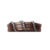•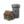+1 直辖上限
•+2 计谋阻力
+0.02 +20
• 始终存在
• 无法篡夺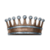•+2 直辖上限
•+4 计谋阻力
•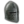+5 骑士数量
•+2 兵士军团数量
+0.05 +40
• 始终存在
• 无法篡夺•+2 直辖上限
•+8 计谋阻力
•+6 骑士数量
•+3 兵士军团数量
•+20 封臣上限
•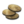+10% 雇佣兵花费
+0.2 +60 250 400 300 375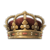•+3 直辖上限
•+16 计谋阻力
•+7 骑士数量
•+4 兵士军团数量
•+40 封臣上限
•+30% 雇佣兵花费
+0.8 +80 500 800 400 1000•+4 直辖上限
•+32 计谋阻力
•+8 骑士数量
•+5 兵士军团数量
•+60 封臣上限
•+60% 雇佣兵花费
+1.6 +100 1000 1200 500 1500

## 法理

• 夺取法理伯爵领宣战理由要求部落时期革新宣战理由
• 夺取法理公国宣战理由要求中世纪早期革新编纂家族编年史
• 夺取法理头衔宣战理由要求中世纪晚期革新合法主权

### 法理转移

• 整个公国（即所有伯爵领）在国王的领地内。
• 公国头衔不存在、被国王持有或被国王的封臣持有。
• 公国不属于耶路撒冷王国。
• 王国是持有者的主头衔。
• 公国和王国的法理领地接壤或最多相隔两片海区。

## 宣称

•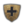强宣称被角色的第一继承人继承，成为弱宣称
•弱宣称不能被角色的第一继承人继承，但如果它被用作宣战理由，而战争无条件和平，该宣称将变成强宣称。
•合法子女获得对其父母所有头衔的潜在宣称，如果父母去世但子女未获得该头衔，对应的潜在宣称将变为强宣称。

• 如果领主的一个头衔至少51%的伯爵领在一个封臣的领地内，则该封臣可以通过“宣称领主头衔”互动花费1500威望获得该头衔的弱宣称
• 使用伪造伯爵领宣称内阁任务将获得目标伯爵领的弱宣称；如果宫廷司祭有高学识，且统治者未拥有伯爵领所属法理公国头衔，将有20%概率触发一个事件，允许角色获得该公国的宣称。
• 宗族族长可以对宗族成员使用“宣称头衔”互动，获得其主头衔的弱宣称。
• 拥有圣许漏洞技能的角色可以使用“购买宣称”互动，获得其他角色主头衔的弱宣称，花费如下：

（注意：如果你角色的主头衔是王国或帝国，你将无法宣称另一个王国/帝国等级的头衔）头衔等级虔诚花费伯爵领 250公国 500王国 1000帝国 2000

### 索取宣称

• 如果信仰拥有男性主导教义，女性的宣称只能用于索取其他女性、儿童或者无能统治者的头衔，反之亦然。
• 只能索取自己、直属封臣和成年廷臣的宣称。

• 对将要剥夺的头衔有宣称。
• 封臣犯下某种严重的罪行。

• 上埃及
• 亚历山大
• 开罗
• 尼罗河三角洲
• 西奈

• 凯鲁万
• 克鲁迈里埃
• 杰里德
• 的黎波里塔尼亚
• 突尼斯

• 康沃尔
• 德赫巴思
• 约克
• 东盎格利亚
• 埃塞克斯
• 圭内斯
• 赫威赛
• 肯特
• 兰开斯特
• 麦西亚
• 诺森布里亚
• 波伊斯
• 威塞克斯

• 奥尔巴尼
• 洛锡安
• 马恩
• 马里
• 斯特拉斯克莱德
• 西部群岛

• 昔兰尼加
• 苏尔特

• 安茹
• 阿基坦
• 阿马尼亚克
• 奥弗涅
• 巴尔
• 贝里
• 波旁
• 布列塔尼
• 勃艮第
• 香槟
• 佛兰德斯
• 加斯科涅
• 朗格多克
• 纽斯特里亚
• 奥尔良
• 普瓦图
• 普罗旺斯
• 萨伏伊
• 图卢兹
• 外汝拉勃艮第
• 上勃艮第
• 瓦卢瓦
• 维耶努瓦

• 阿尔萨斯
• 盎格利亚
• 安哈尔特
• 奥格斯堡
• 奥地利
• 巴伐利亚
• 波西米亚
• 布拉班特
• 卡林西亚
• 库雷齐亚
• 东法兰克尼亚
• 佛兰德斯
• 弗里西亚
• 海尔雷
• 黑森
• 荷兰
• 荷尔斯泰因
• 于利希
• 劳西茨
• 下洛林
• 诺德马克
• 卢森堡
• 迈森
• 摩拉维亚
• 诺德高
• 奥斯特法伦
• 波美拉尼亚
• 东波美拉尼亚
• 萨尔茨堡
• 施泰尔马克
• 图林根
• 蒂罗尔
• 上洛林
• 乌得勒支
• 梅克伦堡
• 西法兰克尼亚
• 威斯特法伦

• 康诺特
• 伦斯特
• 米斯
• 芒斯特
• 阿尔斯特

• 阿尔加维
• 阿拉贡
• 阿斯图里亚斯
• 巴达霍斯
• 巴塞罗那
• 贝雅
• 坎塔布里亚
• 卡斯蒂利亚
• 科英布拉
• 科尔多瓦
• 加利西亚
• 格拉纳达
• 莱昂
• 马略卡
• 穆尔西亚
• 纳瓦拉
• 波图斯卡莱
• 塞维利亚
• 托莱多
• 瓦伦西亚
• 比斯开

• 卡林西亚
• 克罗地亚
• 达尔马提亚
• 杜布罗夫尼克
• 杜克利亚
• 弗留利
• 伊斯特里亚
• 克赖恩
• 马奇瓦
• 拉什卡
• 斯拉沃尼亚
• 上波斯尼亚
• 下波斯尼亚

• 安科纳
• 阿普利亚
• 贝内文托
• 卡拉布里亚
• 卡普阿
• 科西嘉
• 艾米利亚
• 热那亚
• 拉丁姆
• 伦巴第
• 皮埃蒙特
• 比萨
• 罗马涅
• 萨莱诺
• 撒丁尼亚
• 西西里
• 斯波莱托
• 托斯卡纳
• 威尼斯
• 维罗纳

• 阿尔及尔
• 贝贾亚
• 非斯
• 马拉喀什
• 姆扎卜
• 里夫
• 苏斯
• 塔菲拉勒特
• 塔赫尔特
• 特莱姆森
• 扎卜

• 巴格达
• 巴士拉
• 埃德萨
• 克尔曼沙阿
• 美索不达米亚

• 阿勒颇
• 安条克
• 大马士革
• 霍姆斯
• 黎巴嫩
• 外约旦
• 巴勒斯坦
• 约旦

## 头衔列表

•伯爵领列表
•公国列表
•王国列表
•帝国列表

 角色 角色 • 属性 • 特质 • 资源 • 生活方式 • 宗族 • 亲族 • 文化 • 革新 • 传统 • 修正 • 宝物
 领地与管理 领地 • 封臣 • 内阁 • 宫廷 • 谋略 • 政体 • 法律 • 决议 • 头衔 • 男爵领 • 伯爵领 • 朝廷 • 建筑
 战争 战争 • 宣战理由 • 同盟 • 军队 • 受雇军队
 信仰 宗教 • 信仰 • 教义 • 核心教义 • 圣地
 元 模组制作 • 补丁 • 可下载内容 • 开发者日志 • 成就 • 术语